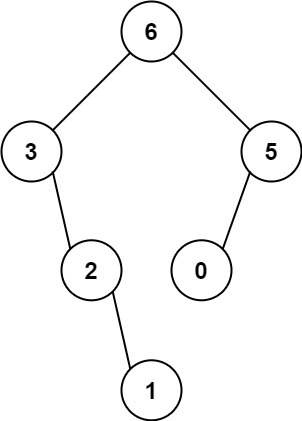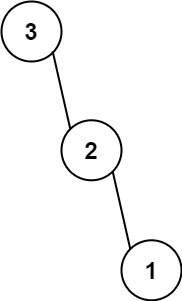# 654. Maximum Binary Tree

Medium
You are given an integer array `nums` with no duplicates. A maximum binary tree can be built recursively from `nums` using the following algorithm:
1. 1.
Create a root node whose value is the maximum value in `nums`.
2. 2.
Recursively build the left subtree on the subarray prefix to the left of the maximum value.
3. 3.
Recursively build the right subtree on the subarray suffix to the right of the maximum value.
Return the maximum binary tree built from `nums`.
Example 1:Input: nums = [3,2,1,6,0,5]
Output: [6,3,5,null,2,0,null,null,1]
Explanation: The recursive calls are as follow:
- The largest value in [3,2,1,6,0,5] is 6. Left prefix is [3,2,1] and right suffix is [0,5].
- The largest value in [3,2,1] is 3. Left prefix is [] and right suffix is [2,1].
- Empty array, so no child.
- The largest value in [2,1] is 2. Left prefix is [] and right suffix is .
- Empty array, so no child.
- Only one element, so child is a node with value 1.
- The largest value in [0,5] is 5. Left prefix is  and right suffix is [].
- Only one element, so child is a node with value 0.
- Empty array, so no child.
Example 2:Input: nums = [3,2,1]
Output: [3,null,2,null,1]
Constraints:
• `1 <= nums.length <= 1000`
• `0 <= nums[i] <= 1000`
• All integers in `nums` are unique.

### 解題

Runtime: 14 ms, faster than 97.40%
Memory Usage: 7.1 MB, less than 68.83%
/**
* Definition for a binary tree node.
* type TreeNode struct {
* Val int
* Left *TreeNode
* Right *TreeNode
* }
*/
func constructMaximumBinaryTree(nums []int) *TreeNode {
if len(nums) == 0 {
return nil
}
if len(nums) == 1 {
return &TreeNode{ Val:nums }
}
maxindex := 0
for i, n := range nums {
if n > nums[maxindex] {
maxindex = i
}
}
return &TreeNode{ Val:nums[maxindex], Left:constructMaximumBinaryTree(nums[:maxindex]), Right: constructMaximumBinaryTree(nums[maxindex + 1:]) }
}